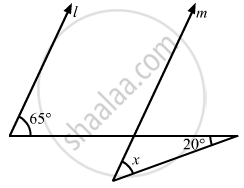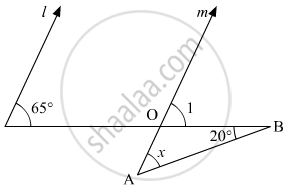# In the Given Figure, If Lines L and M Are Parallel, Then X = - Mathematics

MCQ

In the given figure, if lines l and m are parallel, then x =• 20°

• 45°

• 65°

• 85°

#### Solution

The given figure is as follows:Since, l || m. Thus, 65° angle and ∠1 are corresponding angles.

Therefore,

∠1= 65° (i)

In a triangle, we know that, the exterior angle is equal to the sum of the interior opposite angle.

In ΔAOB:

∠1 = x +20°

x = ∠1 - 20°

From equation (i):

x = 65° - 20°

x = 45°

Concept: Parallel Lines and a Transversal
Is there an error in this question or solution?

#### APPEARS IN

RD Sharma Mathematics for Class 9
Chapter 10 Lines and Angles
Q 20 | Page 55